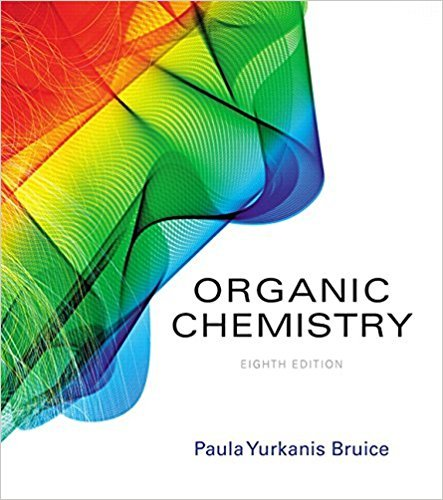×
×

# Solved: Protonated cyclohexylamine has a Ka = 1 * 10-11. Using the same sequence ofISBN: 9780134042282 482

## Solution for problem 95 Chapter 8

Organic Chemistry | 8th Edition

• Textbook Solutions
• 2901 Step-by-step solutions solved by professors and subject experts
• Get 24/7 help from StudySoup virtual teaching assistantsOrganic Chemistry | 8th Edition

4 5 1 293 Reviews
13
2
Problem 95

Protonated cyclohexylamine has a Ka = 1 * 10-11. Using the same sequence of steps as in 94, determine which is a stronger base:cyclohexylamineor aniline.

Step-by-Step Solution:
Step 1 of 3

Week 2 notes  Reaction order o For the reaction The rate law is Where n is equal to the order of the reaction  If n=0 , the reaction is zero order and the rate is independent of the concentration of A  If n=1, the reaction Is first order, and the rate is directly proportional to the concentration of A  If n=2, the reaction is second order,...

Step 2 of 3

Step 3 of 3

##### ISBN: 9780134042282

Unlock Textbook Solution